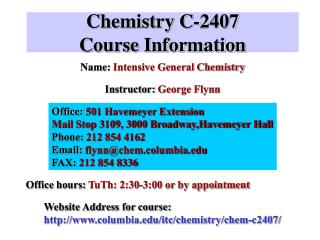DownloadDownload PresentationChemistry C-2407 Course Information

# Chemistry C-2407 Course Information

Télécharger la présentation## Chemistry C-2407 Course Information

- - - - - - - - - - - - - - - - - - - - - - - - - - - E N D - - - - - - - - - - - - - - - - - - - - - - - - - - -
##### Presentation Transcript

1. Chemistry C-2407Course Information Name: Intensive General Chemistry Instructor: George Flynn Office: 501 Havemeyer Extension Mail Stop 3109, 3000 Broadway,Havemeyer Hall Phone: 212 854 4162 Email: flynn@chem.columbia.edu FAX: 212 854 8336 Office hours: TuTh: 2:30-3:00 or by appointment Website Address for course: http://www.columbia.edu/itc/chemistry/chem-c2407/

2. Chemistry C-2407More Course Information Required Recitations: M, 3-5, 6-8; Tu, 3-5; W, 4-6; F, 10-12, 2-4 [Sign up for one only!] These are held in the Chemistry Computer Room Room 211 Havemeyer Telephone Registration: Course # C2409 • Teaching Assistants: Jennifer Inghrim(jai2002@columbia.edu, • mail box #3133, Havemeyer Hall) (854-4964); • Office Hour: Wednesdays 10:00-11:00, Room 343 Havemeyer • and Sean Moran (sdm2007@columbia.edu, • mail box 3139, Havemeyer Hall) (854 8468); • Office Hour: Tuesdays 2:00-3:00, Room 343 Havemeyer First Required Recitation: Tomorrow, Wednesday, September 3, 2003--Bring a blank, unformatted floppy disk!

3. A Little Observation Consider 1 mole of water molecules. H2O has a molecular weight of 18 gm/mole. So, 6.02x1023 molecules weigh 18 gm But, 18 gm of liquid water occupies 18 ml volume (18 cm3) Liquid water is pretty incompressible. So we guess that the water molecules in the liquid are literally in contact with each other. No significant space in between.

4. So the actual volume of one water molecule must be roughly 18 ml/6.02x1023= 3x10-23 ml Contrast this with the volume occupied by a water molecule in the gas phase. To find this number, treat water as an ideal gas at 300 K and use the ideal gas law to compute the volume: pV=nRT with n=1 mole, p= 1 atm. and R=0.082 l-atm/mole-deg V=(1)(.082)(300)/(1)= 24.6 liters Or, the volume occupied per molecule is 24,600ml/6.023x1023= 4.1x10-20 ml Compare! Thus the ratio of the volume occupied by an ideal gas molecule to its actual volume is 24600/18=1400 !!

5. This leads us to conclude that molecules in an ideal gas must be far apart, “rarely” bumping into each other. The volume of an actual molecule is tiny by comparison to the volume occupied at 1 atmosphere and 300K in the gas phase. The picture we walk away with for the gaseous state of a molecule in an ideal gas is one of huge empty spaces between molecules with “rare” collisions. This will allow us to develop a simple MODEL of the gaseous state which provides remarkable insight into the properties of molecules and matter. This MODEL is called the Kinetic Theory of Gases.

6.  v = +10 cm / s, c = 10 cm / s v = -10 cm / s, c = 10 cm / s -x +x 0 2) Particle Momentum is P = mv (not mc). In one dimension:   mgm at c = 10 cm/s m gm at c = 10 cm/s   P = - 10m gm-cm/s P = + 10m gm-cm/s -x +x 0 Kinetic Theory Preliminaries 1) Particle velocity v includes both the speed ( c ) of a particle (cm/s) and its direction. In one dimension: 3) Change in Momentum for an elastic collision: An elastic collision is one where speed is the same before and after the collision:

7. v =+10cm/s -x +x { v = -10cm/s c=10cm/s c=10cm/s Wall

8.  Pinitial = +10m(gm-cm/s) = mv = mc   Pfinal = -10m(gm-cm/s) = mv = -mc    ∆P = Pf - Pi = -mc - (mc) = -2mc [∆ is the symbol for “change”]    ∆Pwall = +2mc ∆Pw + ∆Pm = 0 4) Conservation of Momentum: what particle loses, wall must gain

9. 5) Acceleration a is  (velocity) /  (time). a like v has direction.       a = ∆v / ∆t ( a = dv / dt )   6) Force = F = ma     F = ma = m∆v / ∆t = ∆P / ∆t   F = dP / dt Force is change in momentum with time:

10. Kinetic Theory of Gases Assumptions 1) Particles are point mass atoms (volume = 0) 2) No attractive forces between atoms. Behave independently except for brief moments of collision. Model System A box of volume V with N atoms of mass m all moving with the same speed c. V, N, m, c We wish to calculate the pressure exerted by the gas on the walls of the box:

11. Typical Path for a gas atom or molecule in a box. A A Force of atom impinging on wall creates pressure that we can measure [pV = nRT].

12.   F = ma = m( ∆v )/∆t (∆P)/∆t=(Change in momentum) / (change in time)  Pressure  Force / unit area Thus, we need to find force exerted by atoms on the the wall of the box. Let’s try to calculate the force exerted by the gas on a segment of the box wall having area A. To do this we will make one more simplifying assumption: We assume that all atoms move either along the x, y, or z axes but not at any angle to these axes! (This is a silly assumption and, as we shall see later, causes some errors that we must correct.)

13. Vectors and Vector Components: The Movie Z Y X